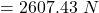## A rifle can shoot a 4.2 g bullet at a speed of 965 m/s. If the work on the bullet is done over a distance of 0.75 m, what is the average for

Question

A rifle can shoot a 4.2 g bullet at a speed of 965 m/s. If the work on the bullet is done over a distance of 0.75 m, what is the average force on the bullet?

in progress 0
1 week 2021-09-05T08:26:56+00:00 1 Answers 0 views 0

The average force on the bullet will be “2607.43 N“.

Explanation:

The given values are:

Mass of the bullet,

m = 4.2 g

Final speed,

v = 965 m/s

Initial speed,

u = 0

Distance,

S = 0.75 m

According to the Energy theorem,

⇒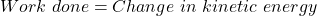⇒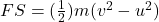On substituting the values, we get

⇒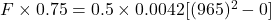⇒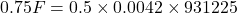⇒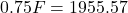⇒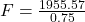⇒